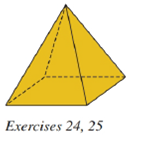Chapter 9.2, Problem 24EElementary Geometry For College St...

7th Edition
Alexander + 2 others
ISBN: 9781337614085

Solutions

Chapter
SectionElementary Geometry For College St...

7th Edition
Alexander + 2 others
ISBN: 9781337614085
Textbook Problem

For the regular square pyramid shown, suppose that the sides of the square base measure 10   m each and that the lateral edges measures 13   m each.a) Find the lateral area L of the pyramid.b) Find the total area A of the pyramid.c) Find the volume V of the pyramid.To determine

(a)

To find:

The lateral area of the pyramid.

Explanation

Given:

A regular square pyramid shown, suppose that the sides of the square base measure 10m each and that the lateral edges measures 13m each.

Properties used:

A pyramid is made by connecting a base to an apex. The base is flat with straight edges, no curves, hence, a polygon and all other faces are triangles.

A regular pyramid is a pyramid whose base is a regular polygon and whose lateral edges are all congruent.

The lateral area L of a regular pyramid with slant height of length l and perimeter P of the base is given by

L=12lP.

According to the Pythagorean theorem, in a right-angled triangle

hypotenuse2=base2+perpendicular2.

The perimeter of the square is four times the side.

Calculation:

From the given figure,

The sides of the square base measure 10m each and that the lateral edges measures 13m each i.e. e=13m

To determine

(b)

To find:

The total area of the pyramid.

To determine

(c)

To find:

The volume of the pyramid.

Still sussing out bartleby?

Check out a sample textbook solution.

See a sample solution

The Solution to Your Study Problems

Bartleby provides explanations to thousands of textbook problems written by our experts, many with advanced degrees!

Get Started

In Problems 31-34, (a) identify d and and (b) write the next three terms. 33.

Mathematical Applications for the Management, Life, and Social Sciences

Fill in each blank: 5yd=ft

Elementary Technical Mathematics

Evaluate the integral. 20. xsin3xdx

Calculus: Early Transcendentals

In Exercises 39-54, simplify the expression. (Assume that x, y, r, s, and t are positive.) 42. 5x6y32x2y7

Applied Calculus for the Managerial, Life, and Social Sciences: A Brief Approach

Find all possible real solutions of each equation in Exercises 3144. x327=0

Finite Mathematics and Applied Calculus (MindTap Course List)

Calculate y'. 46. y=ln|x242x+5|

Single Variable Calculus: Early Transcendentals, Volume I